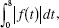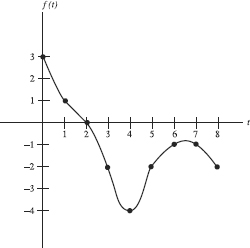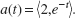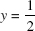# AP Calculus BC Practice Test 30

### Test Information8 questions24 minutes

Calculator Allowed

1. If the half-life of a radioactive substance is 8 years, how long will it take, inyears, for two thirds of the substance to decay?

2. Using the left rectangular method and four subintervals of equal width, estimatewhere f is the function graphed below.3. The area in the first quadrant bounded by the curve with parametric equations x = 2a tan θ and y = 2a cos2 θ, and the lines x = 0 and x = 2a, is equal to

4. The base of a solid is the region bounded by x2 = 4y and the line y = 2, and each plane section perpendicular to the y-axis is a square. The volume of the solid is

5. An object initially at rest at (3,3) moves with accelerationWhere is the object at t = 2?

6. Find the length of the curve y = ln x between the points whereand y = 1.

7. Using the first two terms in the Maclaurin series for y = cos x yields accuracy to within 0.001 over the interval |x| < k when k =

8. After t years, 50e -0.015t pounds of a deposit of a radioactive substance remain. The average amount per yearnot lost by radioactive decay during the second hundred years is